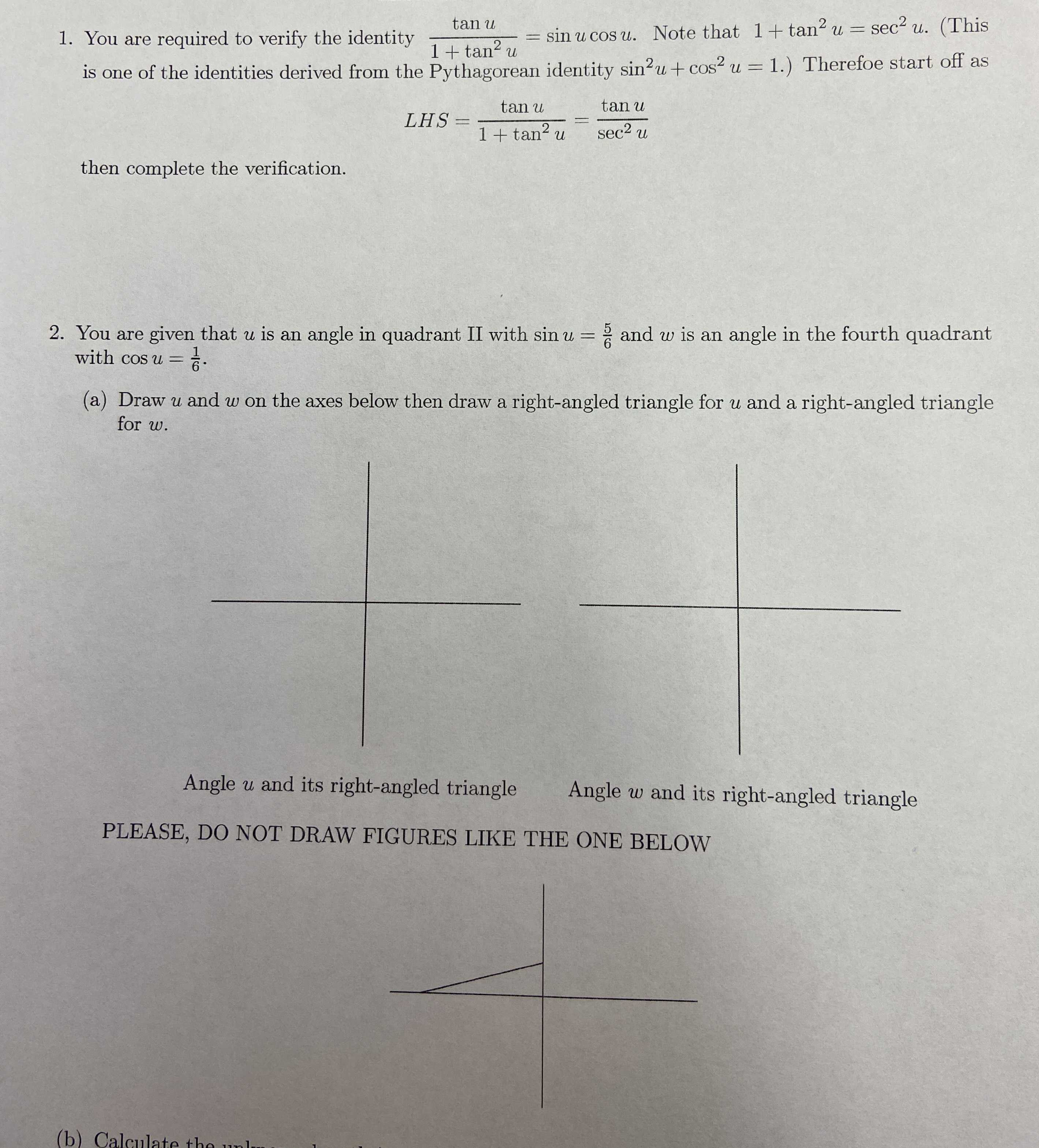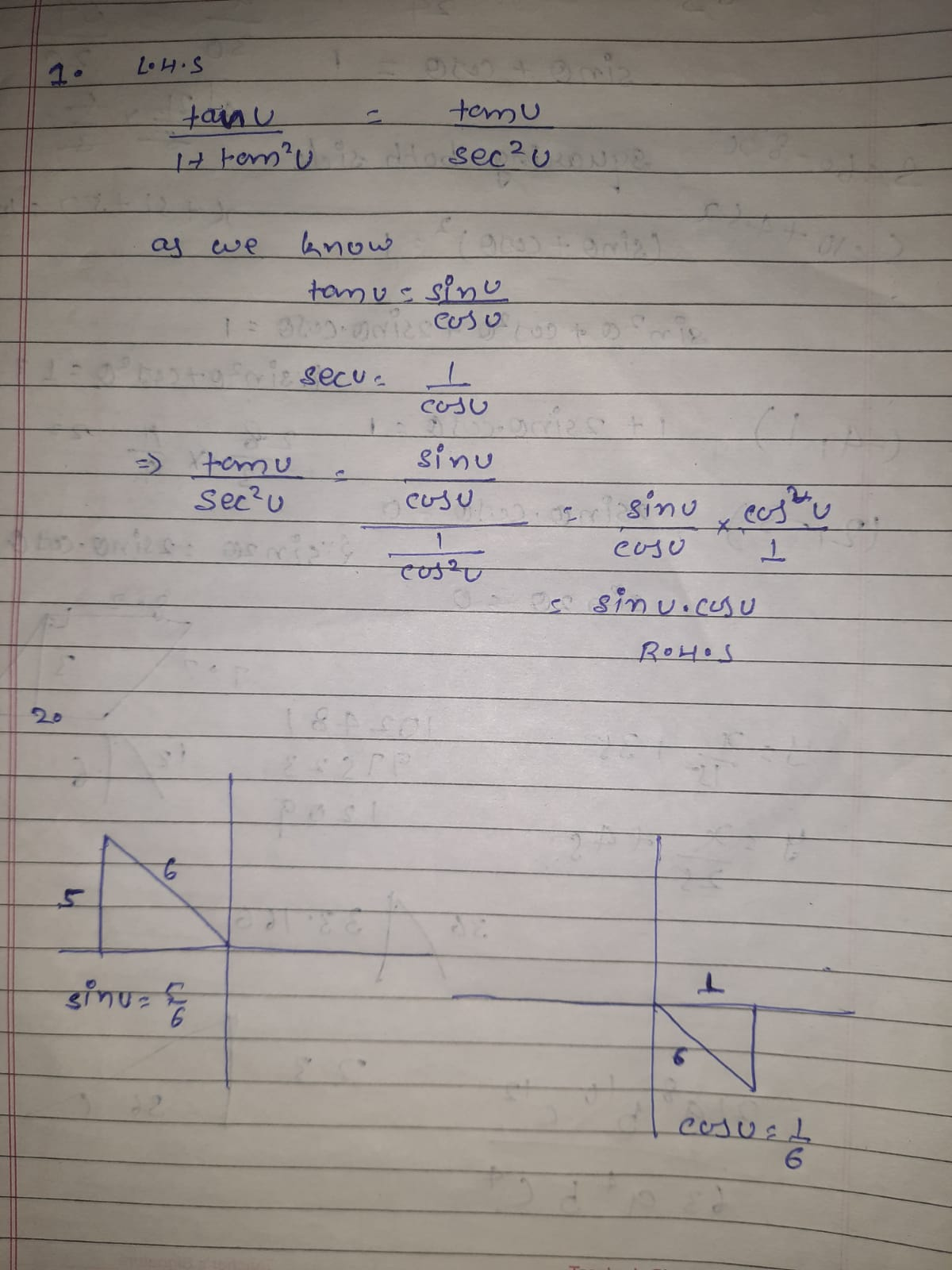### ¿Todavía tienes preguntas de matemáticas?

Pregunte a nuestros tutores expertos
Algebra
Pregunta1. You are required to verify the identity $$\frac { \tan u } { 1 + \tan ^ { 2 } u } = \sin u \cos u .$$ Note that $$1 + \tan ^ { 2 } u = \sec ^ { 2 } u$$ . (This is one of the identities derived from the Pythagorean identity $$\sin ^ { 2 } u + \cos ^ { 2 } u = 1 .$$) Therefore start off as

$$L H S = \frac { \tan u } { 1 + \tan ^ { 2 } u } = \frac { \tan u } { \sec ^ { 2 } u }$$

then complete the verification.

2. You are given that $$u$$ is an angle in quadrant II with $$\sin u = \frac { 5 } { 6 }$$ and $$w$$ is an angle in the fourth quadrant with $$\cos u = \frac { 1 } { 6 }$$ .

(a) Draw $$u$$ and $$w$$ on the axes below then draw a right-angled triangle for $$u$$ and a right-angled triangle for $$w$$ .